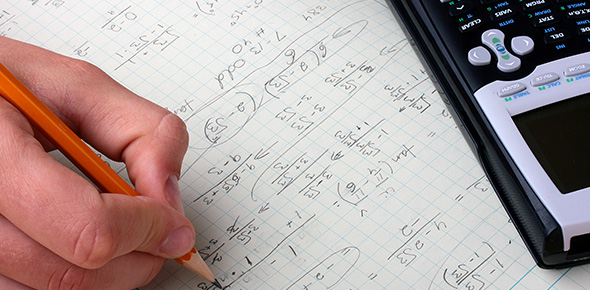# Fundamentals And Functions Of Calculus! Quiz

10 Questions | Attempts: 1613SettingsCalculus is a branch of mathematics that is concerned with limits and with the differentiation and integration of functions which was discovered in the 17th century by I. Newton and G. W. Leibniz. This quiz has been created for students to practice and test their knowledge about fundamentals and functions of calculus. Read the questions carefully and answer. So, let's try out the quiz. All the best!

• 1.
Which of the following can be thought of as a special case of the Mean Value Theorem?
• A.

L'Hopital's rule

• B.

Intermediate Value Theorem

• C.

Rolle's Theorem

• D.

Extreme Value Theorem

• 2.
How many REAL roots does the polynomial x to the fifth +12x-7 have?
• A.

3

• B.

0

• C.

1

• D.

2

• 3.
What is the integral from 0 to Pi over 2 of (Sine of x divided by the sum of Sine of x and Cosine of x)?
• A.

Pi over 2

• B.

Pi over 6

• C.

Pi over 3

• D.

Pi over 4

• 4.
How many roots (real or complex and counting multiple roots the number of times they occur) will a polynomial of degree n have?
• A.

• B.

At least n, but sometimes more

• C.

Exactly n

• D.

At most n, but sometimes less

• 5.
True or False: If two infinitely differentiable functions match in their value and all their derivatives at a point, then the functions must be the same?
• A.

True

• B.

False

• 6.
Are the following statements true or false: A. If a function is differentiable at 0, it is continuous there. B. If a function is differentiable at 0, its derivative is continuous there?
• A.

Neither are true

• B.

B is true, but not A

• C.

A is true, but not B

• D.

Both are true

• 7.
What is the broadest definition of calculus?
• A.

The study of collections of data

• B.

The study of operations and applications

• C.

The study of change

• D.

The study of shape

• 8.
What are the two main branches of calculus?
• A.

Differential and probability

• B.

Differential and integral

• C.

Integral and probability

• D.

Probability and trigonometry

• 9.
Who is widely considered to be the father of calculus (or the father of differential and integral calculus)?
• A.

Both

• B.

Gottfried Wilhelm Leibniz

• C.

Sir Isaac Newton

• D.

Neither

• 10.
When differentiating a function, what does the answer mean? In other words, what does the derivative of a function represent?
• A.

The rate of change of a function at a specific value

• B.

Both

• C.

The slope of the tangent line to a curve

• D.

Neither

## Related TopicsBack to top
×

Wait!
Here's an interesting quiz for you.